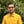Trusted answers to developer questions
Trusted Answers to Developer Questions

Related Tags

c
math
sinh
communitycreator

# What is sinh() in C?Harris Amjad

Grokking Modern System Design Interview for Engineers & Managers

Ace your System Design Interview and take your career to the next level. Learn to handle the design of applications like Netflix, Quora, Facebook, Uber, and many more in a 45-min interview. Learn the RESHADED framework for architecting web-scale applications by determining requirements, constraints, and assumptions before diving into a step-by-step design process.

The sinh() function returns the hyperbolic sine of a number. To be more specific, it returns the hyperbolic sine of a number in the radians float value.

The figure below shows the mathematical representation of the sinh() function.

Figure 1: Mathematical representation of the hyperbolic sine function

The math.h header file is required for this function.

## Syntax

double sinh(double num)


## Parameter

This function requires a number that represents an angle in radians as a parameter.

Use the following formula to convert degrees to radians

radians = degrees * ( PI / 180.0 )


## Return value

sinh() returns the hyperbolic sine of a numberin radians float value that is sent as a parameter.

## Example

#include<stdio.h>
#include<math.h>

int main() {
//positive number in radians
printf("The hyperbolic sine of %lf is %lf \n", 2.3, sinh(2.3));

// negative number in radians
printf("The hyperbolic sine of %lf is %lf \n", -2.3, sinh(-2.3));

//converting the degrees angle into radians and then applying sinh()
// degrees = 45.0
// PI = 3.14159265
// result first converts degrees to radians then apply sinh
double result=sinh(45.0 * (3.14159265 / 180.0));
printf("The hyperbolic sine of %lf is %lf \n", 45.0, result);

}

RELATED TAGS

c
math
sinh
communitycreator

CONTRIBUTORHarris Amjad

Grokking Modern System Design Interview for Engineers & Managers

Ace your System Design Interview and take your career to the next level. Learn to handle the design of applications like Netflix, Quora, Facebook, Uber, and many more in a 45-min interview. Learn the RESHADED framework for architecting web-scale applications by determining requirements, constraints, and assumptions before diving into a step-by-step design process.

Keep Exploring

Learn in-demand tech skills in half the time